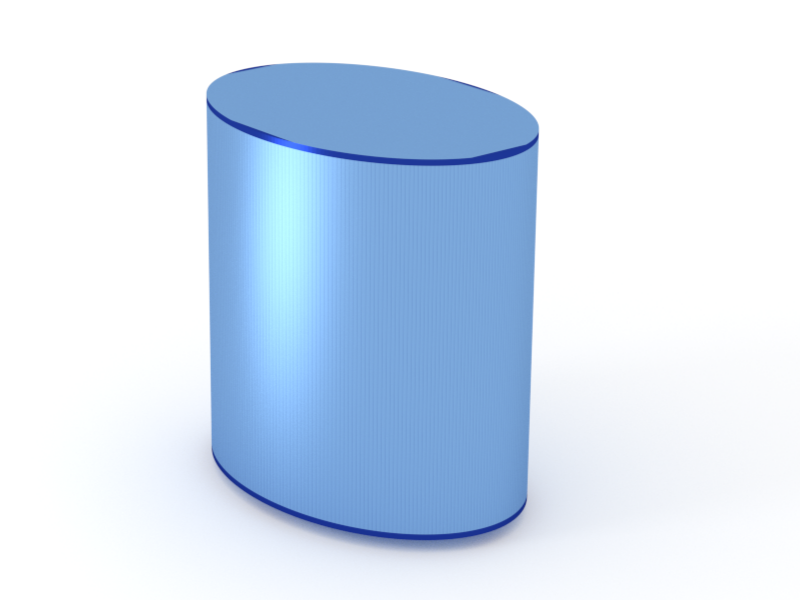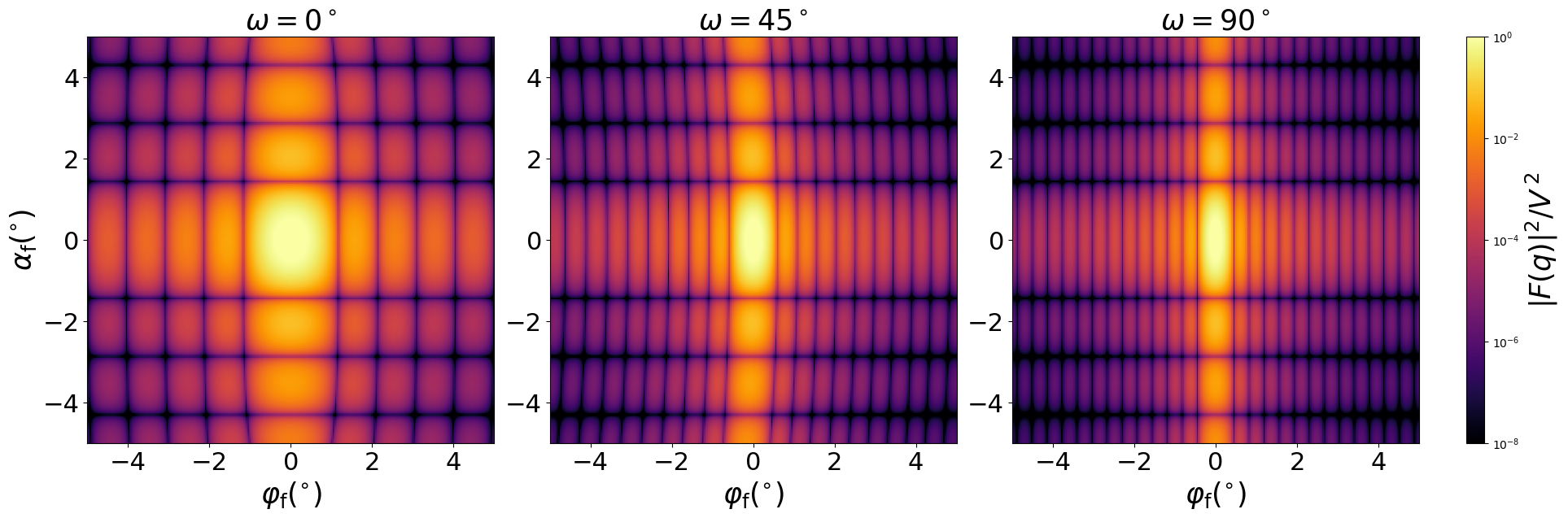### EllipsoidalCylinder

An upright cylinder whose cross section is an ellipse.#### Constructor

EllipsoidalCylinder(R_a, R_b, H)


Parameters:

• R_a, radius $R_{a}$ in $x$ direction
• R_b, radius $R_{b}$ in $y$ direction
• H, height

#### Usage

As for any other Form factor.

#### Implementation

Class EllipsoidalCylinder inherits from the interface class IFormFactor .

Form factor is computed as

$$F(\mathbf{q})=2\pi R_aR_b H \space \exp\Big(iq_z\dfrac{H}{2}\Big) \text{sinc}\Big(q_z\dfrac{H}{2}\Big) \dfrac{J_1(\gamma)}{\gamma} ,$$ with the notation $$\gamma := \sqrt{(q_xR_a)^2 + (q_yR_b)^2}.$$

Volume has been validated against $$V=\pi R_aR_bH.$$

More special:

#### Example

Scattering by uncorrelated, oriented cylinders for horizontal incidence. Rotation around $z$ axis:Generated by Examples/ff/EllipsoidalCylinder.py .

#### History

Agrees with “Ellipsoid” form factor of IsGISAXS [manual, Eq. 2.40, wrongly labeled in Fig. 2.4] and “Ellipsoidal Cylinder” [Renaud 2009, Eq. 224]